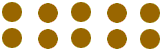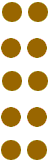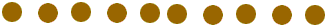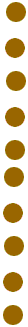Quandaries and Queries How do I create an array of 100 pennies? KATHLEEN Hi Kathleen, I expect that your teacher wants you to put the pennies in a rectangular array. You can do this in many ways. I am going to do this with only 10 pennies and then you can do it with 100. Here is one way to create an array with 10 penniesMathematicians usually describe the size of an array by telling you the number of rows first and then the number of columns, so this is a 2 by 5 array. Here is a 5 by 2 arrayand a 1 by 10 arrayand finally a 10 by 1 arrayThese are all the arrays you can form with 10 pennies. Can you see why? Now you can form some arrays with 100 pennies. Penny Go to Math Central